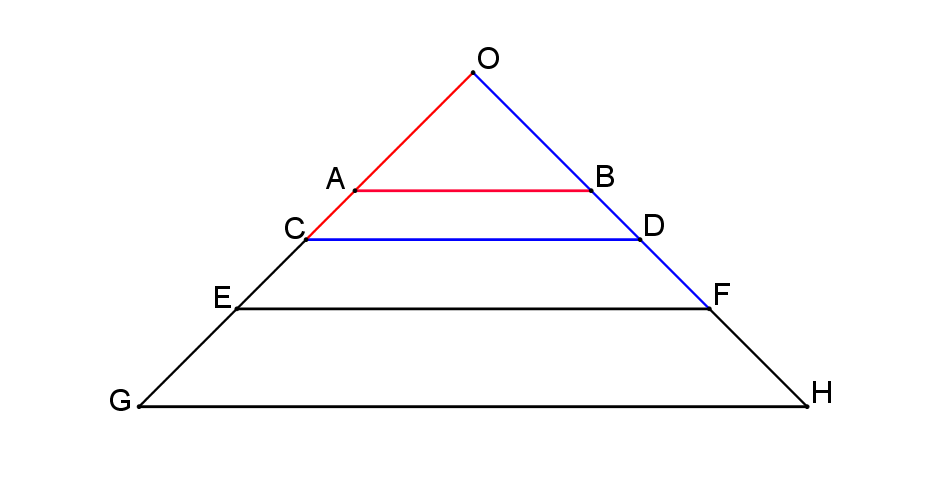#### You may also like### At a Glance

The area of a regular pentagon looks about twice as a big as the pentangle star drawn within it. Is it?### Six Discs

Six circular discs are packed in different-shaped boxes so that the discs touch their neighbours and the sides of the box. Can you put the boxes in order according to the areas of their bases?### Equilateral Areas

ABC and DEF are equilateral triangles of side 3 and 4 respectively. Construct an equilateral triangle whose area is the sum of the area of ABC and DEF.

# Towering Trapeziums

##### Age 14 to 16Challenge Level

$OGH$ is an isosceles right-angled triangle:Lines $AB$, $CD$, $EF$, and $GH$ are parallel.

Suppose the area of the smallest triangle $OAB$ is one square unit.
• If lines $OC$ and $AB$ have the same length, calculate the area of trapezium $ABDC$.
• If lines $OE$ and $CD$ also have the same length, calculate the area of trapezium $CDFE$.
• If lines $OG$ and $EF$ also have the same length, calculate the area of trapezium $EFHG$.
Suppose that the chain of trapezia continued.

What would be the area of the $n^{th}$ trapezium in the chain?